Smartick is a fun way to learn math!# I want to learn about: Number & Operations In Base Ten

Content about numbers for elementary mathematics. Exercises, tutorials, problems, and teaching resources for elementary aged children. Here you will find posts about numbers to make learning mathematics easier and more fun.

May18

## Learn Everything About the Properties of Powers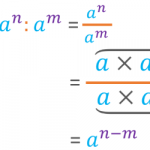In this post, we are going to explain the properties of powers. We will also try to justify the reasoning for following them. Before we begin, let’s remind ourselves of what a power is. What Is a Power? A power is the product of a number multiplied by itself a certain number of times. In […]

Dec23

## Learning Math in the Early Years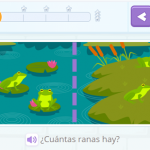When thinking about math in the early years, math for little ones, we contemplate counting, first numbers, and acquiring numerical sequence. And indeed, it is at this stage where we begin to develop the ability to count. But these are not the only math topics that children can learn in the early years. There is […]

Dec02

## How to Find the Absolute Value of a Number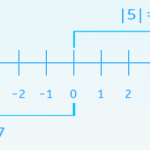In today’s post, we are going to learn how to calculate the absolute value of a number with help from some of the exercises that we have in Smartick. The absolute value of a whole number coincides with its numerical value without having to think about the sign. It is represented with vertical bars around […]

Nov11

## Multiplication of Decimals Using Money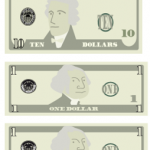Today we are going to better understand the multiplication of decimals by using a very common manipulative material: money. It is going to help us understand why multiplying by 10 moves the decimal position to the right. Equivalence of Bills or Coins with Numbers First, we are going to establish the equivalence of each coin […]

Sep16

## Greater Than and Less Than SymbolsIn order to indicate if a number is greater than or less than another, we use the symbols > and <. For example, 10 is greater than 3, so we write it 10 > 3. If we want to write 2 is less than 6, we write 2 < 6. These symbols look very similar […]

Aug12

## Representing Powers with Rods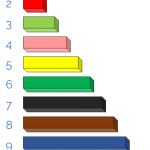In today’s post, I am going to show you how to represent powers with rods. It is fun and easy, you’ll see! We will represent powers to the 2nd and 3rd degree, which are the ones we can reproduce within three dimensions of the physical world. To begin, I will show you a picture of […]

Aug05

## Adding and Subtracting Decimal Numbers Using Money as an Example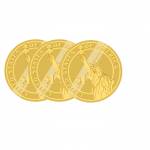To put the addition and subtraction of decimal numbers into practice with money, it is essential to have previous knowledge about decimals and their representation with money. Once we have mastered this knowledge, it’s time to start solving problems. Steps to add and subtract decimal numbers: Place the numbers in columns in such a way […]

Jul08

## Dividing with Decimals Using Money as an Example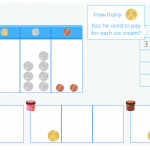In today’s post, you are going to learn how to solve division problems with decimals in the dividend, in other words, dividing a decimal number by a whole number. In order for you to understand the process well, I am going to show you how we explain dividing with decimals in the interactive Smartick tutorial […]

Jul01

## Montessori Cards to Learn Units, Tens and Hundreds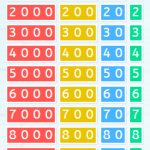The Montessori method was created by Maria Montessori and a large part of her method is facilitating the interaction between children and their reality. In other words, to get them in contact with it by observing, manipulating, and organizing. Ultimately to have them learn from their own experience. Among the main characteristics of the Montessori […]

May06

## Using Money to Help Learn Decimal Numbers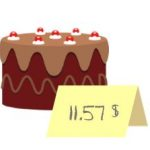Today’s post deals with the day to day use of decimal numbers using money as an example (and to help you better understand it). We are going to start with the basics, reviewing what decimal numbers are and how to read and write them. You can use these resources to refresh your memory: What are […]

Mar11

## Tens and the Decimal Number System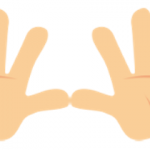Let’s begin this post by defining tens. A ten is a group of ten units. It is the most natural number in our body through the fingers on our hands. If we count them one by one, we can see that we have ten fingers (units), which is the same as saying we have a ten […]